# Equation + addition - math problems

#### Number of problems found: 56

• The ratioThe ratio of two numbers is 4, and their sum is 75. Determine both numbers.
• Two numbers 7The sum of two consecutive even numbers is 30. Find the numbers.
• Lengths of the poolMiguel swam 6 lengths of the pool. Mat swam 3 times as far as Miguel. Lionel swam 1/3 as far as Miguel. How many lengths did Mat swim?
• Solve 2Solve integer equation: a +b+c =30 a, b, c = can be odd natural number from this set (1,3,5,7,9,11,13,15)
• House numbering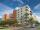The residential house has three entrances numbered even numbers, successive immediately behind. The sum of the two numbers on the outside entrances is 68. Calculate the middle of these three numbers.
• SavingsEva borrowed 1/3 of her savings to her brother, 1/2 of savings spent in the store and 7 euros left. How much did she save?
• Identity123456789 = 100 Use only three plus or minus characters to correct previous identity/equation.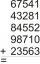Fill letters instead of digits so the indicated sum (equal letters represent equal digits). What number is hidden under the letter J? A A H A H O A H O J -------------------------- 4 3 2 1
• Flowers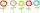The flower has six flowers, and each flower has a number. These are the numbers: 20,40,39,28,8,9. What number will be in the middle of the flower so that the numbers come from the flowers when we subtract and add?
• Summands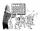Find two summands of the number 42, so that its product is minimized.
• Chocholate pyramid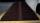How many chocolates are in the third shelf when at the 8th shelf are 41 chocolates in any other shelf is 7 chocolates more the previous shelf.Add marks (+, -, *, /, brackets) to fullfill equations 1 3 6 5 = 10 This is for the 4th grade of the primary school - with no negative numbers yet
• AlgebrogramSolve algebrogram for sum of three numbers: BEK KEMR SOMR ________ HERCI
• Two numbersThe sum of two numbers is 1. Identify this two numbers if you know that the half of first is equal to the third of second number.
• Landlord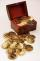Landlord had 49 ducats more than Jurošík. How many ducats Jurošík steal landlord if the Jurošík now 5 ducats more?
• Three ints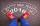The sum of three consecutive integers is 2016. What numbers are they?
• Pupils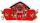Two classes want to collect money. Boys are four-seventh pupils. In time did not pay a quarter of the boys and a sixth of girls, which mean 12 sinners. How many pupils attend these two classes?
• Daughters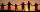The man conducting the census asks a woman to age of three daughters. Woman says when multiply the age getnumber 72; if their ages add up, get a number of our house, as you see. The man says: That is not enough to calculate their ages. She says: my oldest
• Internal angles IST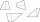Determine internal angles of isosceles trapezium ABCD /a, c are the bases/ and if: alpha:gamma = 1:3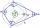What size have angles in quadrilateral (4-gon) if they are in a ratio of 8: 9: 10: 13?